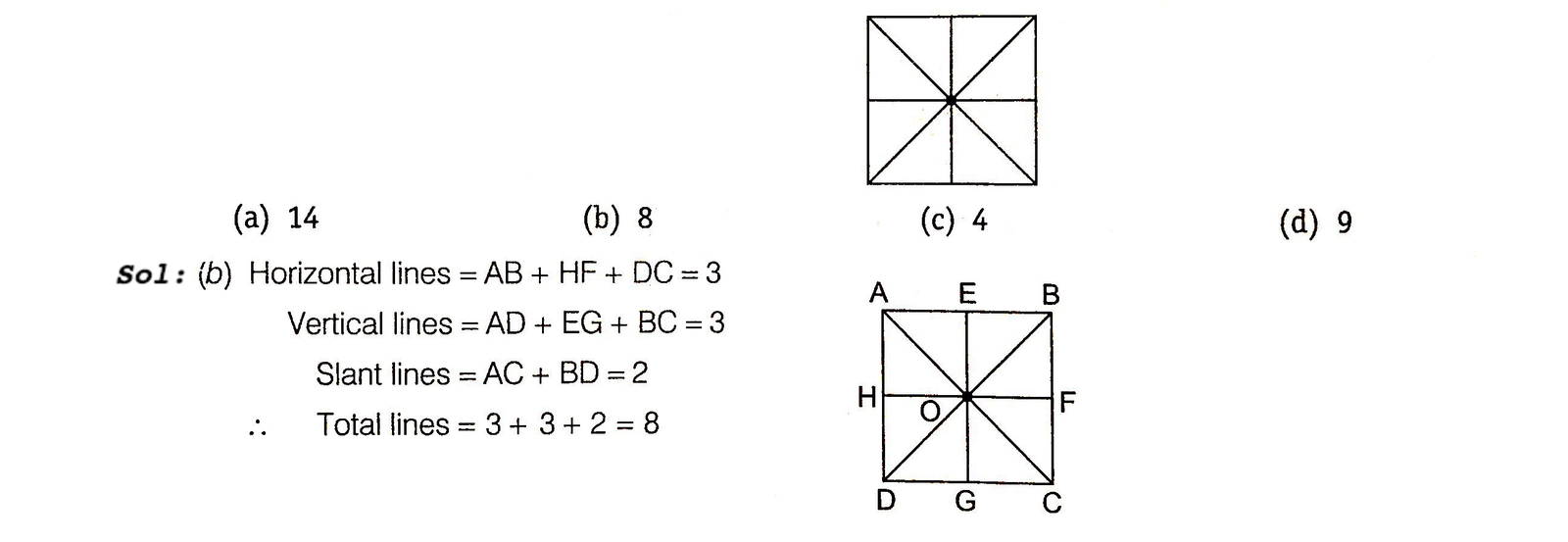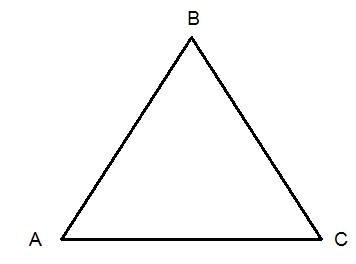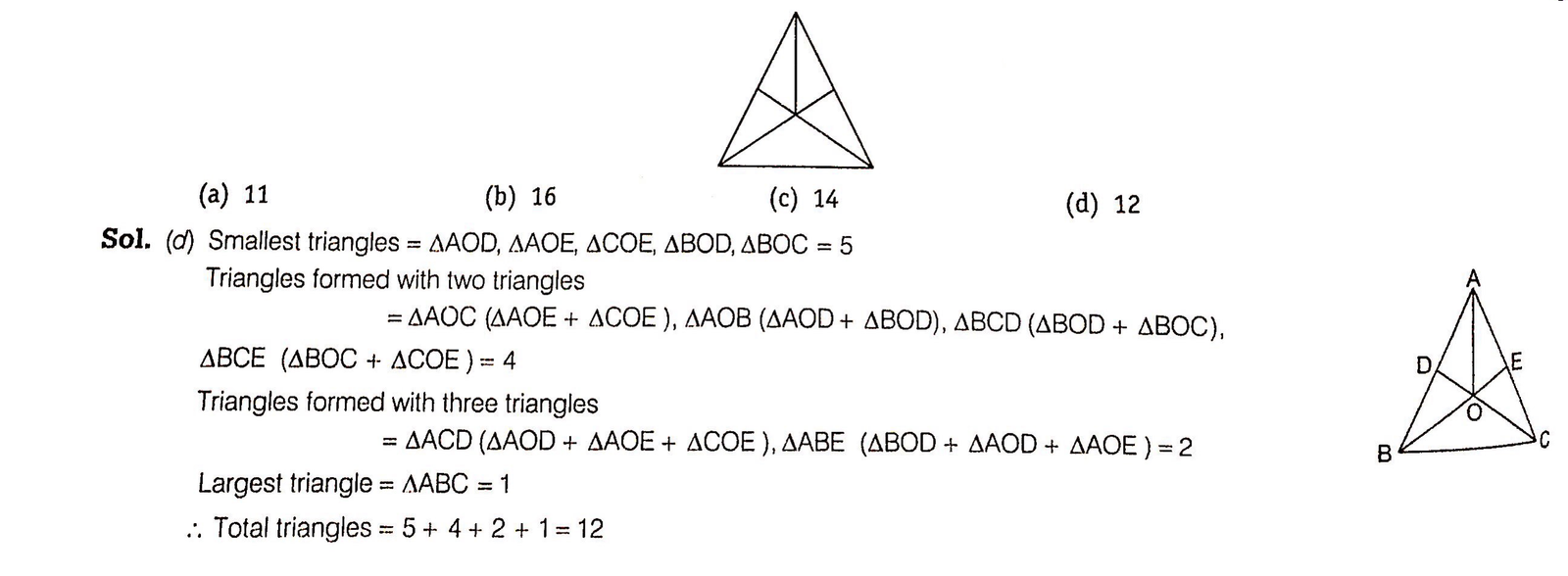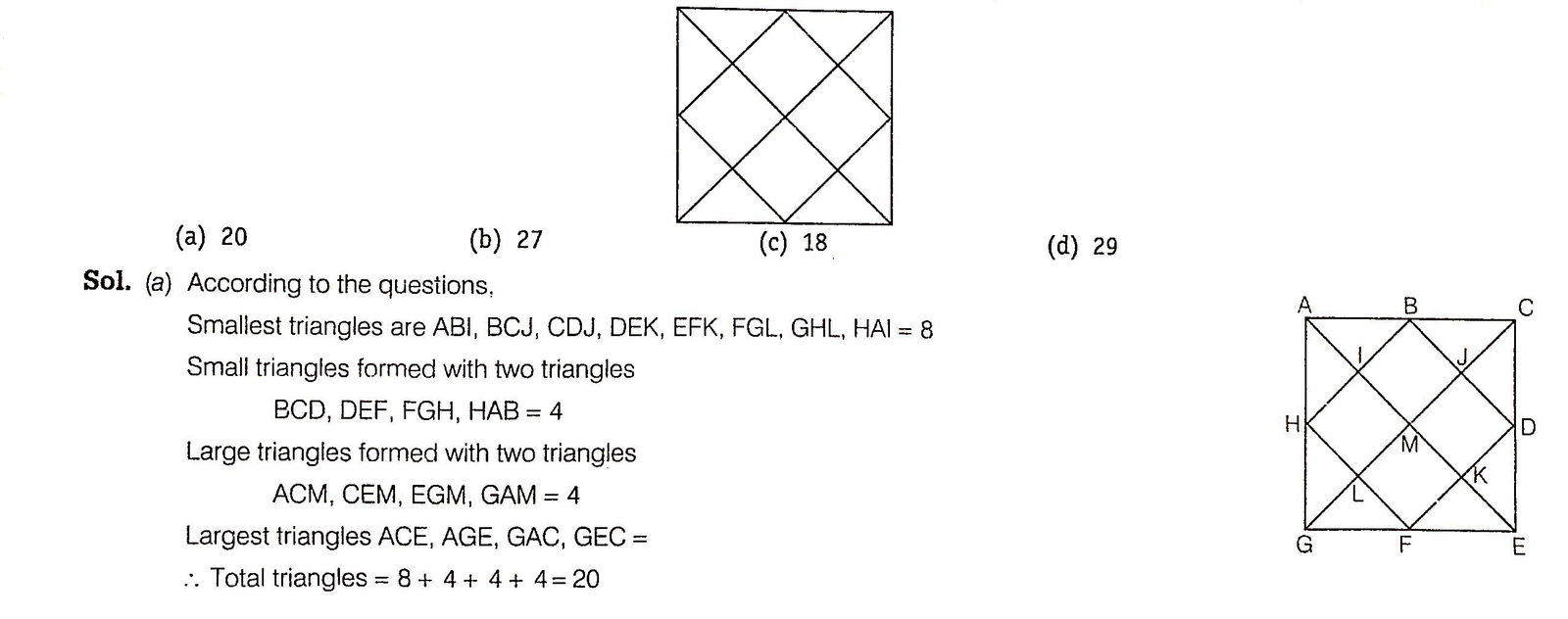# Counting of Figures

Counting of Figures can be explained as the realisation of simple geometrical plane figures from a complex figure.

Different types of questions covered in this chapter are as follows

1. Counting of Straight Lines and Triangles
2. Counting of Quadrilaterals and Polygons
3. Counting of Circles and Colours

This section of non-verbal reasoning is designed to test the analytical ability of the candidate. The figures which are asked for counting can be a straight line, triangle, square, rectangle, polygon etc. To find the accurate answer for these question, firstly, a candidate needs to find the required figures formed by individual section of the given figure, then the figure formed by combination of two figures and so on.

Counting methods of various geometrical figures are covered under different types, which are discussed as below

## Type #1: Counting of Straight Lines and Triangles

In this type of questions, a candidate is required to find out the number of straight lines in the given figure.

A straight line is a set of points that extends endlessly in both the directions.

Some types of straight lines are defined belowCounting Method of Straight Line

Let us consider a line (AB) given

Then, on counting, it will be counted as one line i.e., AB and not as a two straight lines AC and CB.Example 1: How many straight lines are there in the figure given below?Counting of Triangles

In this type of question, a candidate is required to find the number of triangles in the given figures.
A triangle is a closed figure bounded by three side. Here, ABC is a triangle.Counting Method of Triangles

1. Smallest triangles are counted first.
2. At the second step those triangles are counted which are formed with the two triangles and further counting goes on in the same way i.e., triangles formed with three, four, ... triangles are counted one after another.
3. Largest triangle is counted . at the final step.

MIND IT !

Counting can also be done in reverse order i.e., counting in order of largest to smallest.

Example 2: How many triangles are three in the following figure?Example 3: How many triangles are three in the following figure?